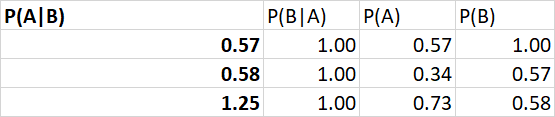# #StackBounty: #bayesian #conditional-probability The probability of a sequence of conditional probabilities with a strict ordering

### Bounty: 50

Let’s assume that we are observing a sequence of choices by an individual. Each choice situation is indexed by $$t = 1,…, T$$ and ordered such that a choice made in $$t = 1$$ precedes a choice made in $$t = 2$$. Furthermore, we cannot observe a choice in $$t = 2$$ unless a particular choice was made in $$t = 1$$. As an observer, I am interested in calculating the probability of observing a particular sequence of choices made by an individual.

The problem can be structured as a decision tree like this:It is obvious from the structure of the problem that observing a choice in $$t = 2$$ is conditional on choosing $$S_1$$ and that observing a choice in $$t = 3$$ is conditional on choosing $$S_1$$ and $$S_2$$ in $$t = 1$$ and $$t = 2$$ respectively. To solve this I thought of using Bayes’ theorem, which states that the conditional probability of $$A$$ given $$B$$ is:

$$P(A|B) = frac{P(B|A)P(A)}{P(B)}$$

To put this in the context of the current problem, let $$A$$ be the probability of observing a choice in $$t$$ and $$B$$ be the probability that you chose $$S$$ in $$t-1$$. Now, $$P(B|A) = 1$$ because the probability that you chose $$S$$ in $$t-1$$ conditional on us observing a choice in period $$t$$ is known with certainty given the strict ordering on $$t$$. This means that $$P(A|B)$$, i.e. the probability of observing a choice conditional on choosing $$S$$ in the previous period, reduces to the ratio $$P(A)/P(B)$$. However, there is no guarantee that $$P(A) < P(B)$$ which means that $$P(A|B)$$ is no longer bound by the unit interval. Now this creates obvious problems.

Let us look at a numerical example to illustrate the practical implications of this. In $$t=1$$ the probability of choosing $$S$$ is .57, in $$t=2$$ it’s 0.34 and in $$t=3$$ it is .73. The probabilities of the others vary, which can happen, but they are specifically chosen extreme here to illustrate the problem.Now, applying Bayes’ theorem above, I get the following:Where $$P(A|B)$$ in period $$t-1$$ is $$P(B)$$ in period $$t$$. Finally, the probability of observing the sequence of choices is the product over the conditional probabilities. However, given the likelihood of getting $$P(A|B) > 1$$, I am concerned that this may not be the correct application of the theorem or even if it is possible in sequence like this. In practice, the sequence of observed choices can be very long.

Get this bounty!!!

This site uses Akismet to reduce spam. Learn how your comment data is processed.